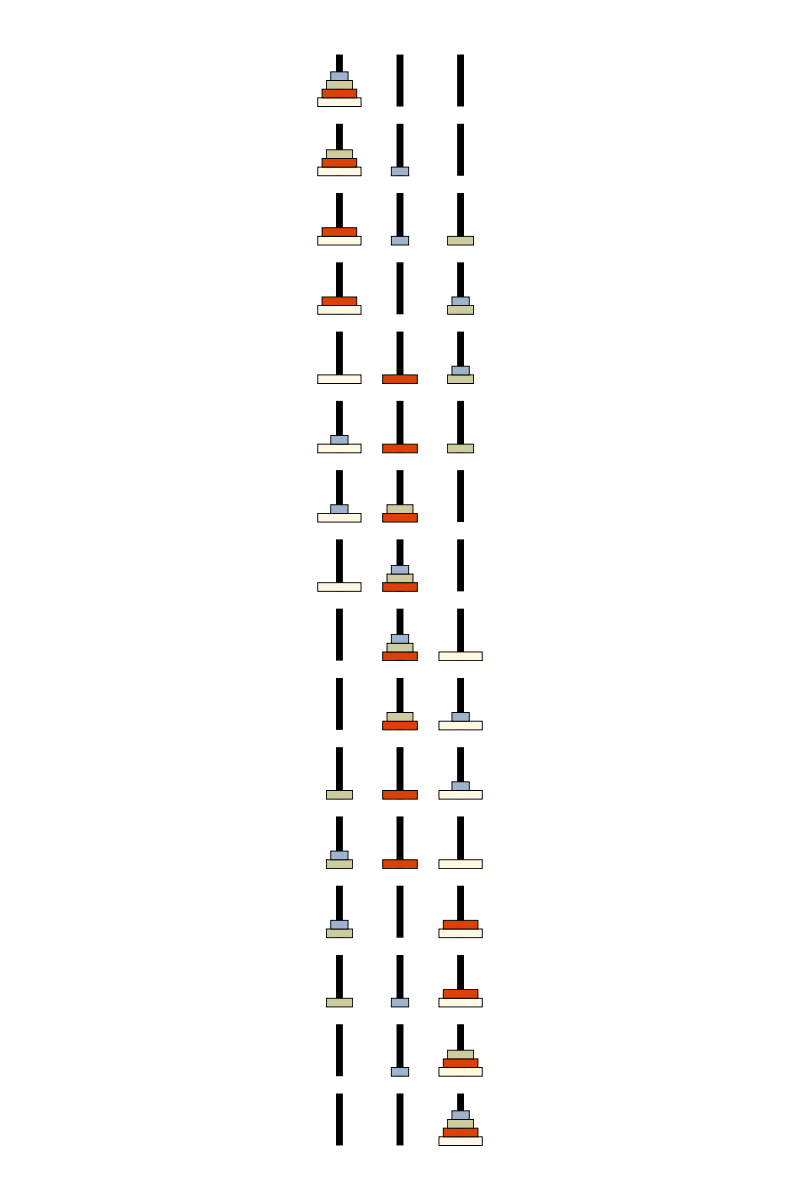Visual solution to the classic Towers of Hanoi puzzle.Author: Brent Yorgey
> import Diagrams.Backend.SVG.CmdLine
> {-# LANGUAGE NoMonomorphismRestriction #-}
> import Diagrams.Prelude
> import Data.List
>
> type Dia = Diagram B

First, some colors for our disks, and types to represent the data structures involved.

> colors = cycle map sRGB24read [ "#9FB4CC", "#CCCC9F", "#DB4105", "#FFF8E3", "#33332D"] > > type Disk = Int > type Stack = [Disk] > type Hanoi = [Stack] > type Move = (Int,Int) To render a single disk, draw a rectangle with width proportional to its disk number, using a color selected from the colors list. > renderDisk :: Disk -> Dia > renderDisk n = rect (fromIntegral n + 2) 1 > # lc black > # lw thin > # fc (colors !! n) To render a stack of disks, just stack their renderings on top of a drawing of a peg. We use alignB to place stack of disks at the bottom of the peg. > renderStack :: Stack -> Dia > renderStack s = disks atop post > where disks = (vcat . map renderDisk s)
>                 # alignB
>         post  = rect 0.8 6
>                 # lw none
>                 # fc black
>                 # alignB

Finally, to render a collection of stacks, lay them out horizontally, using the Distrib method so the pegs end up spaced evenly no matter the width of the disks on any particular peg.

> renderHanoi :: Hanoi -> Dia
> renderHanoi = hcat' (with & catMethod .~ Distrib & sep .~ 7) . map renderStack

Now some code to actually solve the puzzle, generating a list of moves which are then used to simulate the solution and generate a list of configurations.

> solveHanoi :: Int -> [Move]
> solveHanoi n = solveHanoi' n 0 1 2
>   where solveHanoi' 0 _ _ _ = []
>         solveHanoi' n a b c = solveHanoi' (n-1) a c b ++ [(a,c)]
>                               ++ solveHanoi' (n-1) b a c
>
> doMove :: Move -> Hanoi -> Hanoi
> doMove (x,y) h = h''
>   where (d,h')         = removeDisk x h
>         h''            = addDisk y d h'
>         removeDisk x h = (head (h!!x), modList x tail h)
>         addDisk y d    = modList y (d:)
>
> modList i f l  = let (xs,(y:ys)) = splitAt i l in xs ++ (f y : ys)
>
> hanoiSequence :: Int -> [Hanoi]
> hanoiSequence n = scanl (flip ($)) [[0..n-1], [], []] (map doMove (solveHanoi n)) Finally, we render a sequence of configurations representing a solution by laying them out vertically. > renderHanoiSeq :: [Hanoi] -> Dia > renderHanoiSeq = vcat' (with & sep .~2) . map renderHanoi > > example = pad 1.1$ renderHanoiSeq (hanoiSequence 4) # centerXY
> main = mainWith (example :: Diagram B)